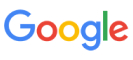New update is available. Click here to update.
Topics

# Search In Rotated Sorted Array

Easy0/40
Average time to solve is 12m+18 more companies

## Problem statement

You have been given a sorted array/list 'arr' consisting of ‘n’ elements. You are also given an integer ‘k’.

Now the array is rotated at some pivot point unknown to you.

For example, if 'arr' = [ 1, 3, 5, 7, 8], then after rotating 'arr' at index 3, the array will be 'arr' = [7, 8, 1, 3, 5].

Now, your task is to find the index at which ‘k’ is present in 'arr'.

Note :
``````1. If ‘k’ is not present in 'arr', then print -1.
2. There are no duplicate elements present in 'arr'.
3. 'arr' can be rotated only in the right direction.
``````

Example:
``````Input: 'arr' = [12, 15, 18, 2, 4] , 'k' = 2

Output: 3

Explanation:
If 'arr' = [12, 15, 18, 2, 4] and 'k' = 2, then the position at which 'k' is present in the array is 3 (0-indexed).
``````

Detailed explanation ( Input/output format, Notes, Images )
Sample Input 1:
``````4 3
8 9 4 5
``````

Sample output 1:
``````-1
``````

Explanation of Sample Output 1:
``````For the test case, 3 is not present in the array. Hence the output will be -1.
``````

Sample Input 2:
``````4 3
2 3 5 8
``````

Sample output 2:
``````1
``````

Expected Time Complexity:
``````Try to do this in O(log(n)).
``````

Constraints:
``````1 <= n <= 10^5
0 <= k <= 10^9
0 <= arr[i] <= 10^9

Time Limit: 1 second
``````Console